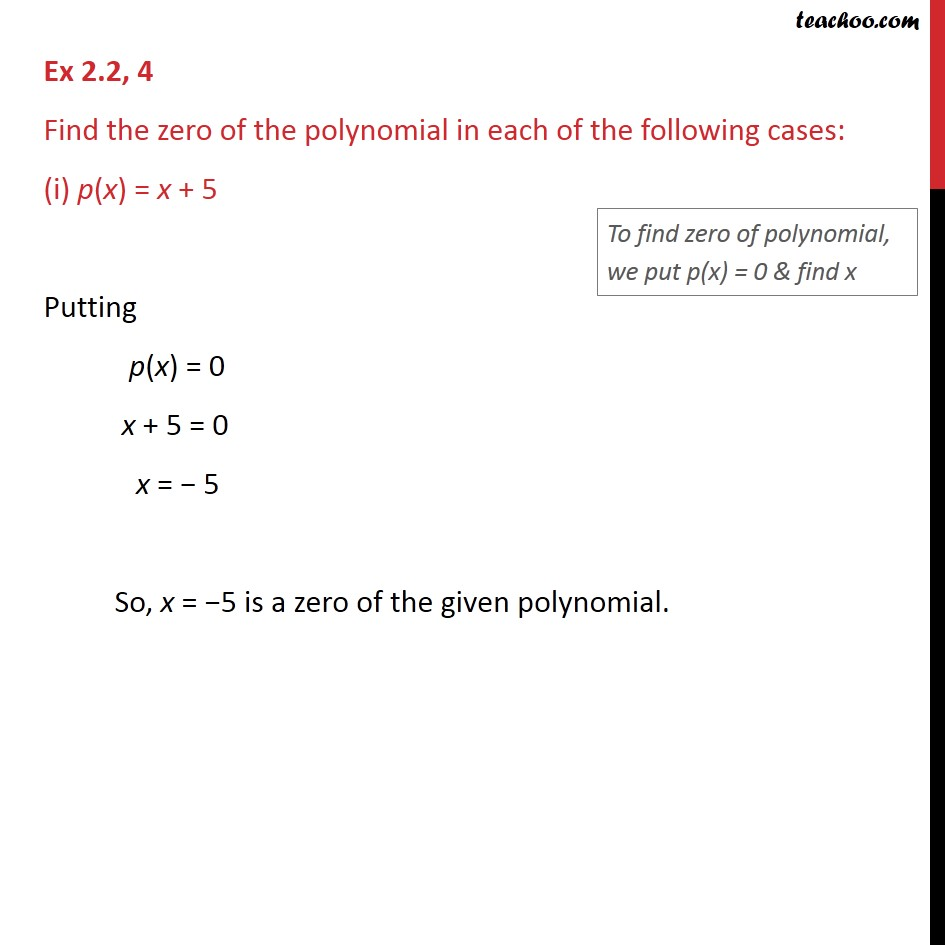1. Chapter 2 Class 9 Polynomials (Term 2)
2. Concept wise
3. Finding Zeroes of a polynomial

Transcript

Ex 2.2, 4 Find the zero of the polynomial in each of the following cases: (i) p(x) = x + 5 Putting p(x) = 0 x + 5 = 0 x = − 5 So, x = −5 is a zero of the given polynomial.